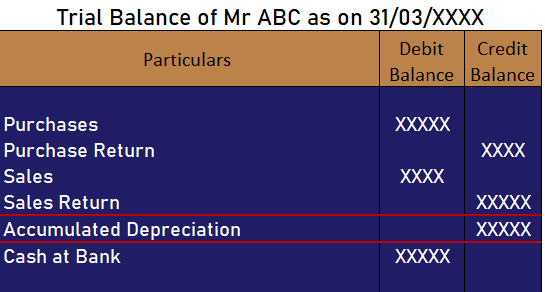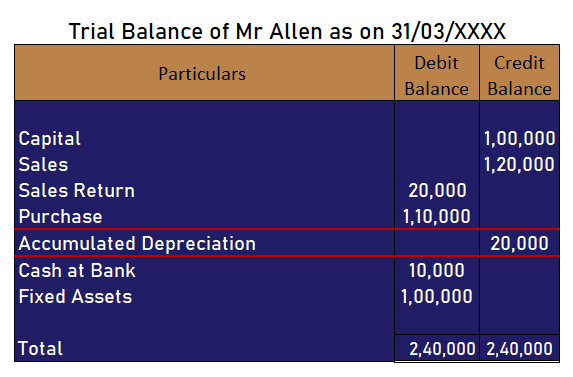## Is accumulated depreciation an asset or liability?

1. Accumulated Depreciation Depreciation is a wear and tear of an asset due to efflux of time and various other factors. It's basically an allocation of the cost of a tangible asset over its useful life. Accumulated depreciation is the total depreciation charged on an asset until a specified date. ItsRead more

## Accumulated Depreciation

Depreciation is a wear and tear of an asset due to efflux of time and various other factors. It’s basically an allocation of the cost of a tangible asset over its useful life.

Accumulated depreciation is the total depreciation charged on an asset until a specified date. Its a contra asset account. And since its a contra asset account it reduces the balance of an asset i.e reduces debit balance and therefore has a credit balance.

## Accumulated Depreciation is an Asset or a Liability?

Well if you ask me I would say that its neither an asset nor a liability.

Reasons to justify the above statement:

Why is it not an asset?

Assets are the resources held by an entity so that it could provide some economic value for the entity. But, in the case of accumulated depreciation, it does not generate any economic benefit for an entity rather it indicated that a certain sum of economic benefit has already been availed.

Why is it not a liability?

A liability is an obligation of an entity for making payment at a specified future date to a third party. Here, accumulated depreciation does not represent an obligation of an entity rather it is maintained just for the purpose of record-keeping.

## Conclusion

According to the reasons mentioned above it can neither be called as an asset nor a liability. This would be the correct answer to this question.

But still, if you have to compulsorily classify the same as an asset or a liability I would definitely not classify as a liability as it would not ensure fair representation of the financial statements since then it would be considered an obligation made to a third party which is not the case here.

See less
• 4
• Share
Share

## How is accumulated depreciation shown in trial balance?

1. Accumulated Depreciation in a Trial Balance The accumulated depreciation is shown as a "credit item" in the trial balance. Accumulated depreciation is nothing but the sum total of depreciation charged until a specified date. Since in every reporting period, a part of a fixed asset is written off i.eRead more

## Accumulated Depreciation in a Trial Balance

The accumulated depreciation is shown as a “credit item” in the trial balance. Accumulated depreciation is nothing but the sum total of depreciation charged until a specified date. Since in every reporting period, a part of a fixed asset is written off i.e depreciated such accumulated depreciation has a credit balance.

You should have a glance at the image of an extract of the trial balance given- below it will definitely answer your question in a more effective way.## Accumulated Depreciation

As mentioned earlier the accumulated depreciation is the sum total of depreciation that an entity has expensed in its profit and loss statement till that date. It’s basically a contra asset account as it reduces the balance in the asset account.

Illustrative Example,

Prepare a trial balance of Mr Allen on the basis of given heads of accounts –

 Particulars Amount Capital 1,00,000 Sales 1,20,000 Purchases 1,10,000 Sales Return 20,000 Fixed Assets 1,00,000 Cash at bank 10,000 Accumulated Depreciation 20,000

Solution :Aastha Mehta.

See less
• 1
• Share
Share